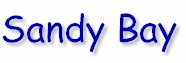Weather CenterWeather Calculators - Also see Distance Calculators

Temperature Conversion
Enter a number, then click on the appropriate button to see the result.

 Fahrenheit ( o F ) Celsius (°C)
 Celsius ( o C ) Fahrenheit (o F)

Windchill Calculation

 Temp (o F) Wind Speed (mph)
Wind chill =
 Temp (o C) Wind Speed (mph)
Wind chill =

Relative Humidity Calculation

 Temp (°F ) Dew Point (°F)
Relative Humidity =
 Temp (°C) Dew Point (°C )
Relative Humidity =

Heat Index Calculation

Valid entries are, air temperatures greater than 80 °F ( 27 °C ), dew point temperatures greater than 65 °F ( 12 °C ), and relative humidities higher than 40 percent.

 Temp (°F ) Dew Point (°F)
RH =
 Temp (°C) Dew Point (°C )
RH =
 Temp (°F ) Relative Humidity (%)
Heat Index =
Dew Point =
 Temp (°C) Relative Humidity (%)
Heat Index =
Dew Point =

#### Convert from one speed to another

1 knot = 1 nautical mile per hour = 6076 feet per hour = 1.15078 mph
1 mph = 1 mile per hour = 5280 feet per hour = 0.86898 knots per hour

Type a value in one box and click here to see the results

 Kilometers per hour Miles per hour Knots

Original weather calculators  java script by Ron Moore of the Birmingham, AL, National Weather Service